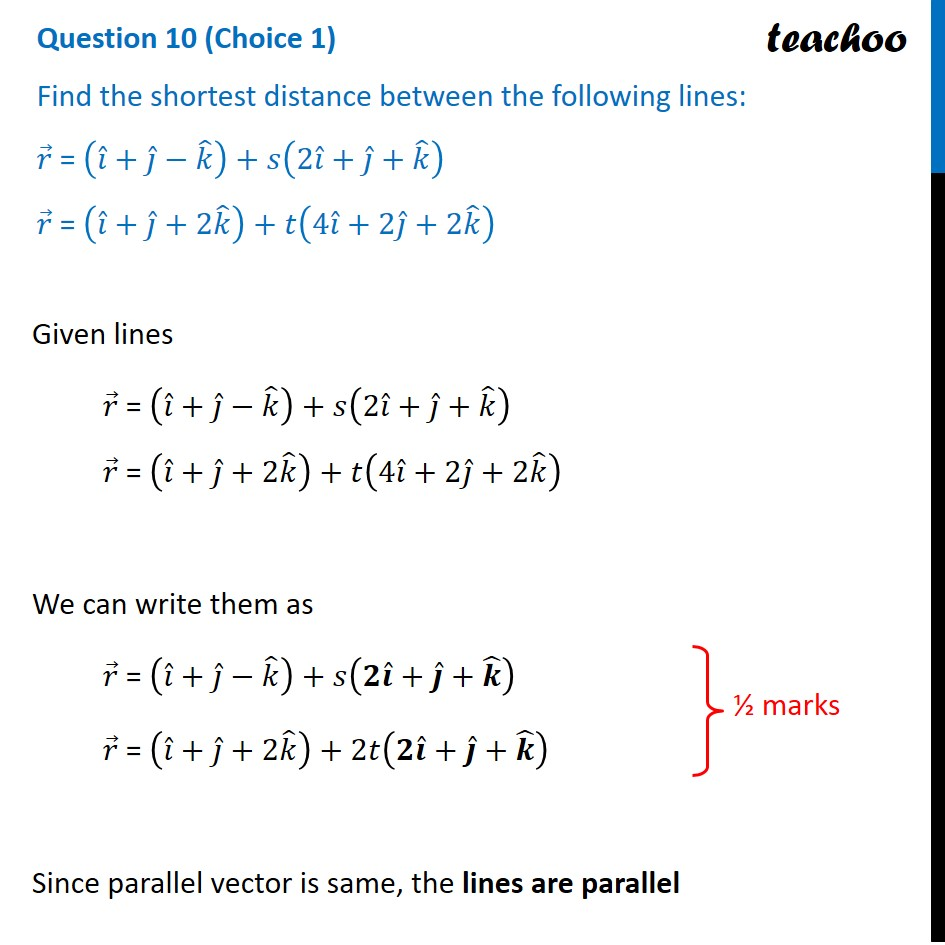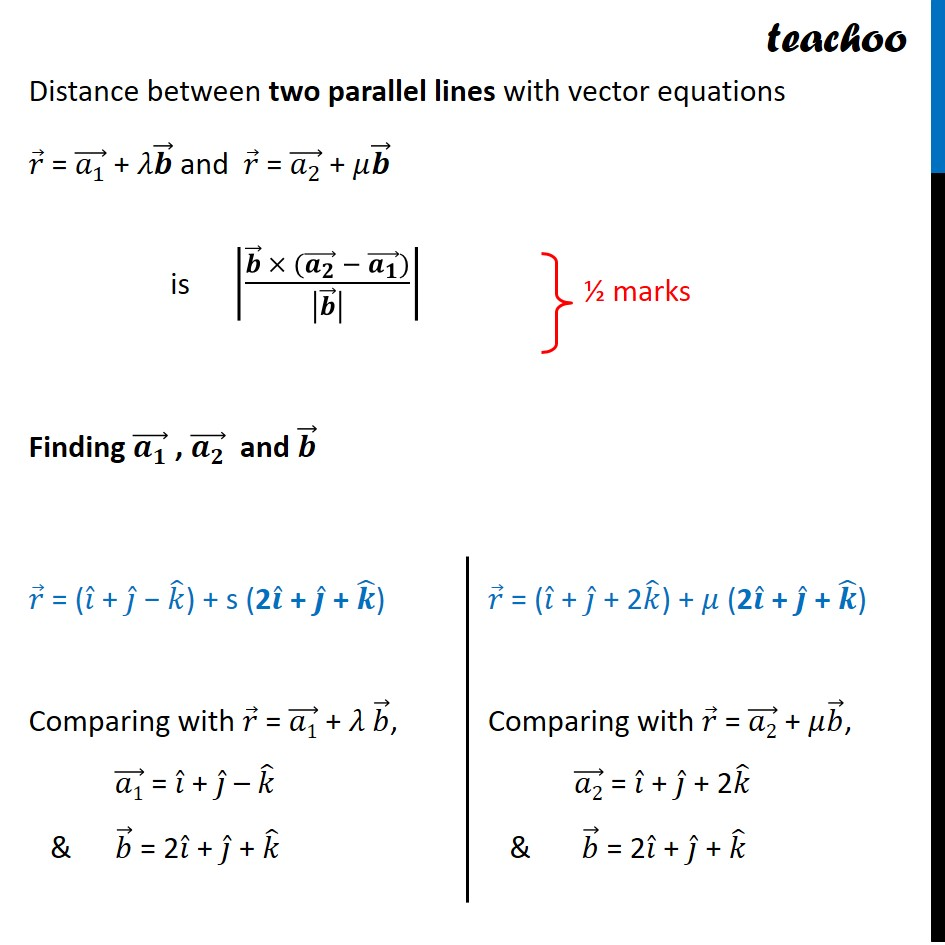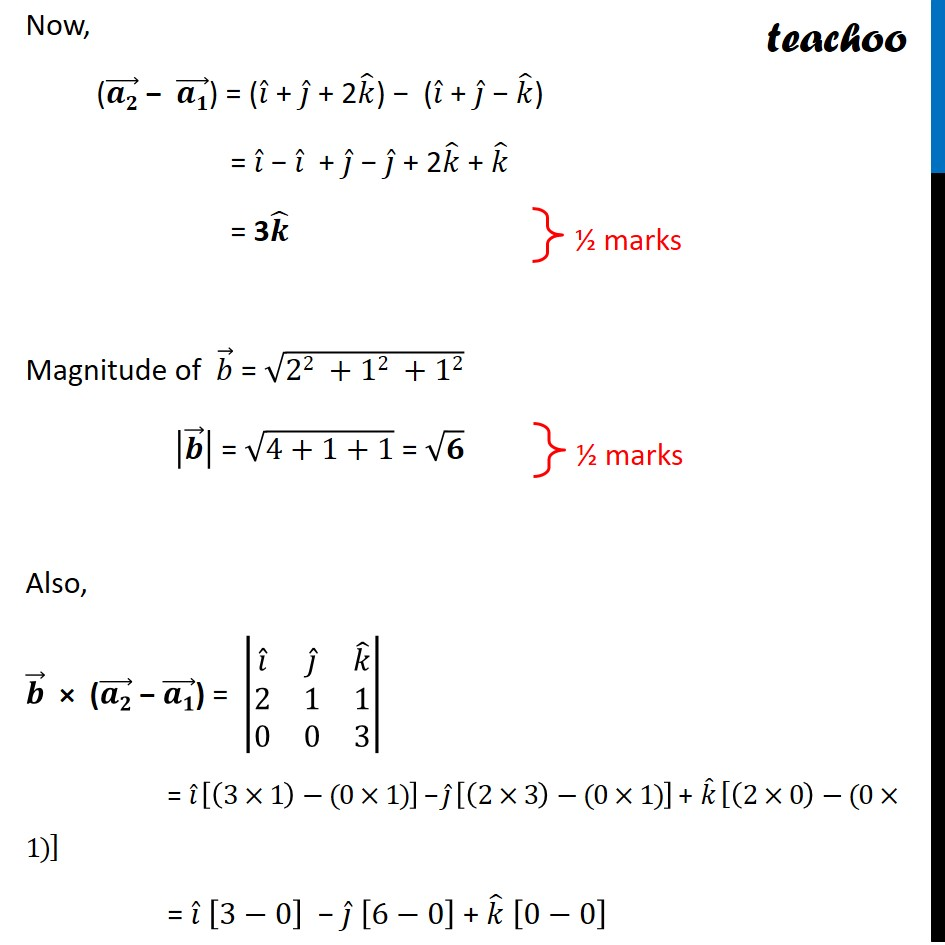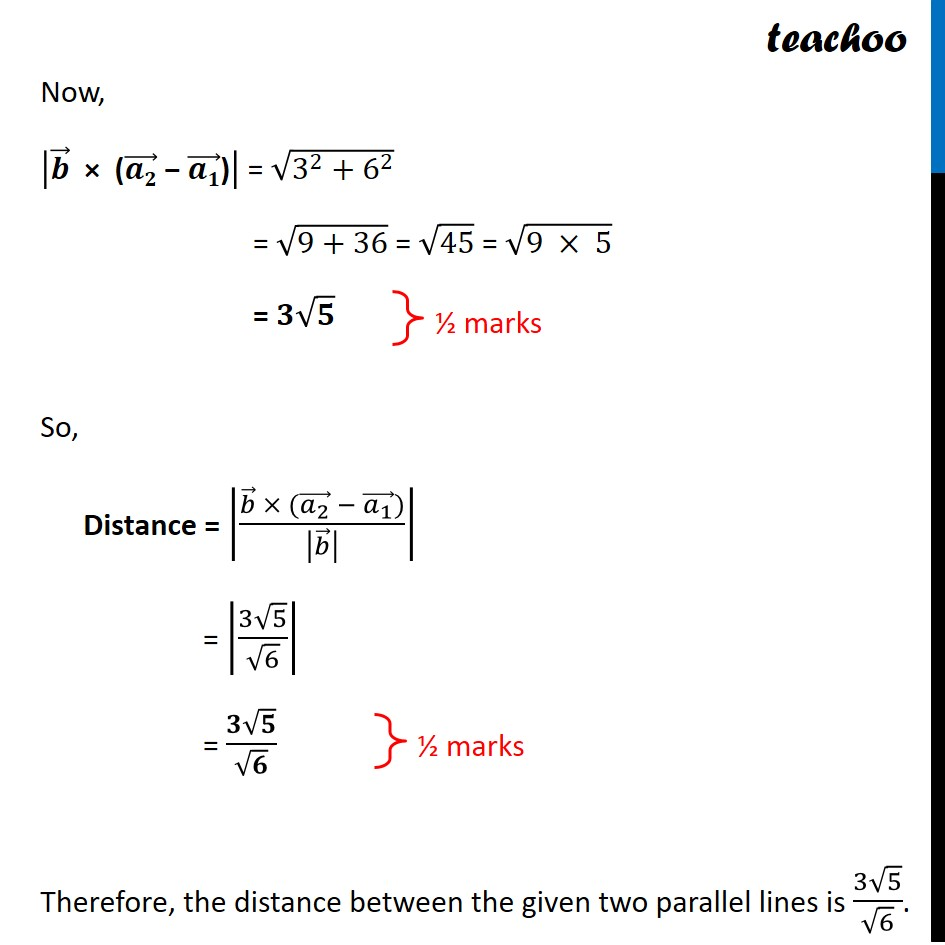CBSE Class 12 Sample Paper for 2022 Boards (For Term 2)

Class 12
Solutions of Sample Papers and Past Year Papers - for Class 12 Boards

## r ⃗ = (i ̂+j ̂+k ̂ )+t(4i ̂+(2j) ̂+2k ̂ )

This question is similar to Example 12 - Chapter 11 Class 12 - Three Dimensional GeometryLearn in your speed, with individual attention - Teachoo Maths 1-on-1 Class

### Transcript

Question 10 (Choice 1) Find the shortest distance between the following lines: 𝑟 ⃗ = (𝑖 ̂+𝑗 ̂−𝑘 ̂ )+𝑠(2𝑖 ̂+𝑗 ̂+𝑘 ̂ ) 𝑟 ⃗ = (𝑖 ̂+𝑗 ̂+2𝑘 ̂ )+𝑡(4𝑖 ̂+2𝑗 ̂+2𝑘 ̂ ) Given lines 𝑟 ⃗ = (𝑖 ̂+𝑗 ̂−𝑘 ̂ )+𝑠(2𝑖 ̂+𝑗 ̂+𝑘 ̂ ) 𝑟 ⃗ = (𝑖 ̂+𝑗 ̂+2𝑘 ̂ )+𝑡(4𝑖 ̂+2𝑗 ̂+2𝑘 ̂ ) We can write them as 𝑟 ⃗ = (𝑖 ̂+𝑗 ̂−𝑘 ̂ )+𝑠(𝟐𝒊 ̂+𝒋 ̂+𝒌 ̂ ) 𝑟 ⃗ = (𝑖 ̂+𝑗 ̂+2𝑘 ̂ )+2𝑡(𝟐𝒊 ̂+𝒋 ̂+𝒌 ̂ ) Since parallel vector is same, the lines are parallel Distance between two parallel lines with vector equations 𝑟 ⃗ = (𝑎_1 ) ⃗ + 𝜆𝒃 ⃗ and 𝑟 ⃗ = (𝑎_2 ) ⃗ + 𝜇𝒃 ⃗ is |(𝒃 ⃗ × ((𝒂_𝟐 ) ⃗ − (𝒂_𝟏 ) ⃗))/|𝒃 ⃗ | | Finding (𝒂_𝟏 ) ⃗ , (𝒂_𝟐 ) ⃗ and 𝒃 ⃗ 𝑟 ⃗ = (𝑖 ̂ + 𝑗 ̂ − 𝑘 ̂) + s (2𝒊 ̂ + 𝒋 ̂ + 𝒌 ̂) Comparing with 𝑟 ⃗ = (𝑎1) ⃗ + 𝜆 𝑏 ⃗, (𝑎1) ⃗ = 𝑖 ̂ + 𝑗 ̂ – 𝑘 ̂ & 𝑏 ⃗ = 2𝑖 ̂ + 𝑗 ̂ + 𝑘 ̂ 𝑟 ⃗ = (𝑖 ̂ + 𝑗 ̂ + 2𝑘 ̂) + 𝜇 (2𝒊 ̂ + 𝒋 ̂ + 𝒌 ̂) Comparing with 𝑟 ⃗ = (𝑎2) ⃗ + 𝜇𝑏 ⃗, (𝑎2) ⃗ = 𝑖 ̂ + 𝑗 ̂ + 2𝑘 ̂ & 𝑏 ⃗ = 2𝑖 ̂ + 𝑗 ̂ + 𝑘 ̂ Now, ((𝒂𝟐) ⃗ − (𝒂𝟏) ⃗) = (𝑖 ̂ + 𝑗 ̂ + 2𝑘 ̂) − (𝑖 ̂ + 𝑗 ̂ − 𝑘 ̂) = 𝑖 ̂ − 𝑖 ̂ + 𝑗 ̂ − 𝑗 ̂ + 2𝑘 ̂ + 𝑘 ̂ = 3𝒌 ̂ Magnitude of 𝑏 ⃗ = √(22 +12 +12) |𝒃 ⃗ | = √(4+1+1) = √𝟔 Also, 𝒃 ⃗ × ((𝒂𝟐) ⃗ − (𝒂𝟏) ⃗) = |■8(𝑖 ̂&𝑗 ̂&𝑘 ̂@2&1&1@0&0&3)| = 𝑖 ̂ [(3×1)−(0×1)] − 𝑗 ̂ [(2×3)−(0×1)] + 𝑘 ̂ [(2×0)−(0×1)] = 𝑖 ̂ [3−0] − 𝑗 ̂ [6−0] + 𝑘 ̂ [0−0] = 𝟑𝒊 ̂ − 6𝒋 ̂Now, |𝒃 ⃗" × (" (𝒂𝟐) ⃗" − " (𝒂𝟏) ⃗")" | = √(3^2+6^2 ) = √(9+36) = √45 = √(9 × 5) = 𝟑√𝟓 So, Distance = |(𝑏 ⃗ × ((𝑎_2 ) ⃗ − (𝑎_1 ) ⃗))/|𝑏 ⃗ | | = |(3√5)/√6| = (𝟑√𝟓)/√𝟔 Therefore, the distance between the given two parallel lines is (3√5)/√6.